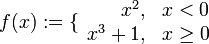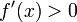# Difference between revisions of "First derivative test fails for function that is discontinuous at the critical point"

Jump to: navigation, search

## Statement

### What fails

Suppose$f$ is a function and$c$ is a point in the interior of the domain of$f$. Suppose$f$ is not continuous at$c$. Then, we cannot use the first derivative test directly, and naively looking at the statement of the test could yield incorrect conclusions.

## Example

Consider the function:$f(x) := \lbrace \begin{array}{rl} x^2, & x < 0 \\ x^3 + 1, & x\ge 0 \\\end{array}$

We see that:$f'(x) = \lbrace \begin{array}{rl} 2x, & x < 0 \\ 3x^2, & x > 0 \\\end{array}$

Note that$f'$ is not defined at 0 because$f$ is not continuous at zero.

We thus see that$f'(x) < 0$ for$x$ to the immediate left of 0 and$f'(x) > 0$ for$x$ to the immediate right of 0. Thus,$f'$ is changing sign from negative to positive. A naive application of the first derivative test suggests that$f$ has a local minimum at zero. However, this is not correct. The reason is that the function jumps upward at 0, hence it does not attain a local minimum from the left at zero.